## Relational-Algebra

 Question 1
Consider the following relations P(X,Y,Z), Q(X,Y,T) and R(Y,V).How many tuples will be returned by the following relational algebra query?
x(P.Y=R.Y ∧ R.V=V2)(P × R)) - ∏x(Q.Y=R.Y ∧ Q.T>2)(Q × R))
 A 0 B 1 C 2 D 3
Database-Management-System       Relational-Algebra       GATE 2019       Video-Explanation
Question 1 Explanation:
σ(P.Y = R.Y ∧ R.V = V2)(P × R)x(P.Y = R.Y ∧ R.V = V2)(P × R))σ(Q.Y = R.Y ∧ Q.T>2)(Q × R)x(Q.Y = R.Y ∧ Q.T>2)(Q × R))x(P.Y = R.Y ∧ R.V = V2)(P × R)) - ∏x(Q.Y = R.Y ∧ Q.T>2)(Q × R))Question 2
Consider the relations r(A, B) and s(B, C), where s.B is a primary key and r.B is a foreign key referencing s.B. Consider the query
Q: r⋈(σ<B<5 (s))
Let LOJ denote the natural left outer-join operation. Assume that r and s contain no null values.
Which one of the following is NOT equivalent to Q?
 A σB<5 (r ⨝ s) B σB<5 (r LOJ s) C r LOJ (σB<5(s)) D σB<5(r) LOJ s
Database-Management-System       Relational-Algebra       GATE 2018       Video-Explanation
Question 2 Explanation:
Consider the following relations r(A, B) and S(B, C), where S.B is a primary key and r.B is a foreign key referencing S.B
Consider the following tables without NULL values.Q: r⨝(σB<5(S))
The result of σB<5(S) is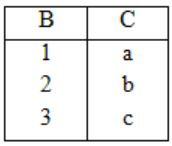The result of σB<5(S) isOption (A):
The result of r⨝S isThe result of σB<5(r⨝S) isOption (B):
The result of r LOJ S isThe result of σB<5(r LOJ S) isOption (C):
The result of σB<5(S) isNow, the result of r LOJ(σB<5(S))Option (D):
The result of σB<5(r) isNow, the result of σB<5(r) LOJ S isTherefore, from the output of above four options, the results of options, the results of options (A), (B) and (D) are equivalent to Q.
 Question 3
Consider a database that has the relation schemas EMP(EmpId, EmpName, DeptId), and DEPT(DeptName, DeptId). Note that the DeptId can be permitted to a NULL in the relation EMP. Consider the following queries on the database expressed in tuple relational calculus.
(I) {t│∃u ∈ EMP(t[EmpName] = u[EmpName] ∧ ∀v ∈ DEPT(t[DeptId] ≠ v[DeptId]))}
(II) {t│∃u ∈ EMP(t[EmpName] = u[EmpName] ∧ ∃v ∈ DEPT(t[DeptId] ≠ v[DeptId]))}
(III) {t│∃u ∈ EMP(t[EmpName] = u[EmpName] ∧ ∃v ∈ DEPT(t[DeptId] = v[DeptId]))}
Which of the above queries are safe?
 A (I) and (II) only B (I) and (III) only C (II) and (III) only D (I), (II) and (III)
Database-Management-System       Relational-Algebra       GATE 2017 [Set-1]       Video-Explanation
Question 3 Explanation:
An expression is said to be safe, if it yield a finite number of tuples, otherwise unsafe.
(I) Gives EmpNames who do not belong to any Department. So, it is going to be a finite number of tuples as a result.
(II) Gives EmpNames who do not belong to some Department. This is also going to have finite number of tuples.
(III) Gives EmpNames who do not belong to same Department. This one will also give finite number of tuples.
All the expressions I, II and III are giving finite number of tuples. So, all are safe.
 Question 4

Consider a database that has the relation schema CR(StudentName, CourseName). An instance of the schema CR is as given below.The following query is made on the database.

```         T1 ← πCourseName(σStudentName='SA'(CR))
T2 ← CR ÷ T1
```

The number of rows in T2 is ____________.

 A 4 B 5 C 6 D 7
Database-Management-System       Relational-Algebra       GATE 2017 [Set-1]       Video-Explanation
Question 4 Explanation:
(1) T1 ← πCourseNameStudentName = 'SA'(CR))
The σStudentName = 'SA'(CR) will produce the following⇾ The result of T1 ← πCourseNameStudentName='SA'(CR)) is(2) T2 ← CR÷T1
⇾ We see that SA is enrolled for CA, CB and CC.
⇾ T2 will give the StudentNames those who have enrolled for all the CA, C, CC courses. So, the following Students are enrolled for the given 3 courses.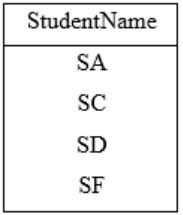⇾ So, the output of T2 will have 4 rows.
 Question 5

Consider two relations R1(A,B) with the tuples (1,5), (3,7) and R2(A,C) = (1,7), (4,9). Assume that R(A,B,C) is the full natural outer join of R1 and R2. Consider the following tuples of the form (A,B,C): a = (1.5,null), b = (1,null,7), c = (3,null,9), d = (4,7,null), e = (1,5,7), f = (3,7,null), g = (4,null,9). Which one of the following statements is correct?

 A R contains a,b,e,f,g but not c, d. B R contains all of a,b,c,d,e,f,g C R contains e,f,g but not a,b D R contains e but not f,g
Database-Management-System       Relational-Algebra       GATE 2015 [Set-2]
Question 5 Explanation:⋆ So, from the above resultant table, R contains e, f, g only but not a, b.
 Question 6

Suppose R1(A, B) and R2(C, D) are two relation schemas. Let r1 and r2 be the corresponding relation instances. B is a foreign key that refers to C in R2. If data in r1 and r2 satisfy referential integrity constraints, which of the following is ALWAYS TRUE?

 A ∏B (r1) - ∏C (r2) = ∅ B ∏C (r2) - ∏B (r1) = ∅ C ∏B (r1) = ∏C (r2) D ∏B (r1) - ∏C (r2) ≠ ∅
Database-Management-System       Relational-Algebra       GATE 2012
Question 6 Explanation:
Referential integrity means, all the values in foreign key should be present in primary key.
So we can say that r2(C) is the superset of r1(B).
So (subset - superset) is always empty.
 Question 7

Consider the following relations A, B, C.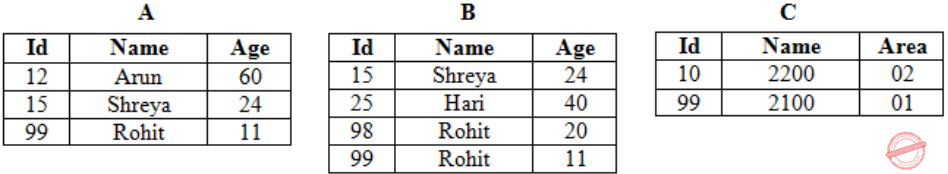How many tuples does the result of the following relational algebra expression contain? Assume that the schema of AUB is the same as that of A.

`(AUB)⋈A.Id>40∨C.Id<15 C`
 A 7 B 4 C 5 D 9
Database-Management-System       Relational-Algebra       GATE 2012
Question 7 Explanation:Performs the cross product and selects the tuples whose A∙Id is either greater than 40 or C∙Id is less than 15. It yields:Question 8

Which of the following functional dependencies hold for relations R(A, B, C) and S(B, D, E):

```B -> A
A -> C```

The relation R contains 200 tuples and the rel ation S contains 100 tuples. What is the maximum number of tuples possible in the natural join of R and S (R natural join S)

 A 100 B 200 C 300 D 2000
Database-Management-System       Relational-Algebra       GATE 2010
Question 8 Explanation:
Given
R(A, B, C) – 200 tuples
S(B, D, E) – 100 tuples
FD’s:
B → A
A → C
― ‘B’ is primary key for R and foreign key of S from the given FDs.
― Maximum tuples in natural join of R and S is min(200, 100) = 100.
 Question 9

Let R and S be two relations with the following schema

R(P,Q,R1,R2,R3)
S(P,Q,S1,S2)

Where {P, Q} is the key for both schemas. Which of the following queries are equivalent?

I. ΠP(R ⨝ S)
II. ΠP(R) ⨝ ΠP(S)
III. ΠPP,Q(R) ∩ ΠP,Q(S))
IV. ΠPP,Q(R) - (ΠP,Q(R) - ΠP,Q(S)))
 A Only I and II B Only I and III C Only I, II and III D Only I, III and IV
Database-Management-System       Relational-Algebra       GATE 2008
Question 9 Explanation:
Natural join is based on the common columns of the two tables.
We have two common columns in 'R' and 'S' which are 'P' and 'Q'.
(I) Both P and Q are used while doing the join, i.e., both P and Q are used to filter.
(II) Q is not used here for filtering. Natural join is done on all P's from R and all P's from S. So different from option (I).
(III) Through venn diagram it can be proved that A∩B = A - (A-B).
So through above formula we can say that (III) and (IV) are equivalent.
So, finally (I), (III) and (IV) are equivalent.
 Question 10

Information about a collection of students is given by the relation studinfo(studId, name, sex). The relation enroll(studId, courseId) gives which student has enrolled for (or taken) that course(s). Assume that every course is taken by at least one male and at least one female student. What does the following relational algebra expression represent?

ΠcourseId((ΠstudIdsex="female"(studInfo))×ΠcourseId(enroll))-enroll)
 A Courses in which all the female students are enrolled. B Courses in which a proper subset of female students are enrolled. C Courses in which only male students are enrolled. D None of the above
Database-Management-System       Relational-Algebra       GATE 2007
Question 10 Explanation:
Option A: It is not result the all the female students who are enrolled. So option A is false.
Option B: Yes, True. It selects the proper subset of female students which are enrolled because in the expression we are performing the Cartesian product.
Option C: False. It doesn’t shows (or) display the males students who are enrolled.
 Question 11

Let r be a relation instance with schema R = (A, B, C, D). We define r1 = ΠA,B,C(R) and r2 = ΠA,D(R). Let s = r1*r2 where * denotes natural join. Given that the decomposition of r into r1 and r2 is lossy, which one of the following is TRUE?

 A s ⊂ r B r ∪ s = r C r ⊂ s D r * s = s
Database-Management-System       Relational-Algebra       GATE 2005
Question 11 Explanation:
Let us consider an example:
Table r: R(A, B, C, D)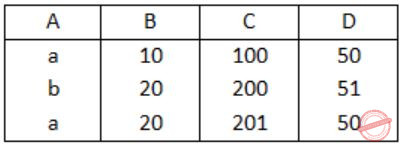Table r1: ΠA,B,C(R)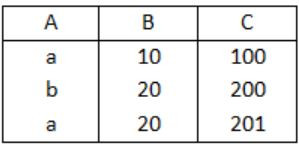Table r2: ΠA,D(R)S = r1 * r2 (* denotes natural join)
Table S:Table r ⊂ Table S
⇒ r ⊂ S
 Question 12

Let R1(A,B,C) and R2(D,E) be two relation schema, where the primary keys are shown underlined, and let C be a foreign key in R1 referring to R2. Suppose there is no violation of the above referential integrity constraint in the corresponding relation instances r1 and r2. Which one of the following relational algebra expressions would necessarily produce an empty relation?

 A ΠD(r2) - ΠC(r1) B ΠC(r1) - ΠD(r2) C ΠD(r1⨝C≠Dr2) D ΠC(r1⨝C=Dr2)
Database-Management-System       Relational-Algebra       GATE 2004
Question 12 Explanation:
C be a foreign key in R1 referring to R2.
→ Based on referral integrity C is subset of values in R2 then,
ΠC(r1) - ΠD(r2) results empty relation.
 Question 13

Consider the following relation schema pertaining to a students database:

```   Student (rollno, name, address)
Enroll (rollno, courseno, coursename) ```

Where the primary keys are shown underlined. The number of tuples in the Student and Enroll tables are 120 and 8 respectively. What are the maximum and minimum number of tuples that can be present in (Student * Enroll), where '*' denotes natural join ?

 A 8, 8 B 120, 8 C 960, 8 D 960, 120
Database-Management-System       Relational-Algebra       GATE 2004
Question 13 Explanation:
Natural join is a JOIN operation that creates an implicit join cause for you based on common columns in the two tables being joined.
→ In the question only enroll Id's are same with the student table.
→ The no. of minimum and maximum tuples is same i.e., 8, 8.
 Question 14

Consider the relation Student (name, sex, marks), where the primary key is shown underlined, pertaining to students in a class that has at least one boy and one girl. What does the following relational algebra expression produce? (Note: p is the rename operator).The condition in join is "(sex = female ^ x = male ^ marks ≤ m)"

 A names of girl students with the highest marks B names of girl students with more marks than some boy student C names of girl students with marks not less than some boy students D names of girl students with more marks than all the boy students
Database-Management-System       Relational-Algebra       GATE 2004
Question 14 Explanation:
In the operator '-' between the two subexpression gives the names of all female students whose marks are greater than the all the male students of the class.
 Question 15

With regard to the expressive power of the formal relational query languages, which of the following statements is true?

 A Relational algebra is more powerful than relational calculus. B Relational algebra has the same power as relational calculus. C Relational algebra has the same power as safe relational calculus. D None of the above.
Database-Management-System       Relational-Algebra       GATE 2002
Question 15 Explanation:
Relational algebra has the same power as safe relational calculus.
A query can be formulated in safe Relational Calculus if and only if it can be formulated in Relational Algebra.
 Question 16

Suppose the adjacency relation of vertices in a graph is represented in a table Adj(X,Y). Which of the following queries cannot be expressed by a relational algebra expression of constant length?

 A List of all vertices adjacent to a given vertex B List all vertices which have self loops C List all vertices which belong to cycles of less than three vertices D List all vertices reachable from a given vertex
Database-Management-System       Relational-Algebra       GATE 2001
Question 16 Explanation:
(a) Finding a adjacency vertex for the given vertex is too simple i.e., Adj(X,Y).
(b) Finding a self loop is also simple (Oop(X,X))
(c) If a → b, b → c then c!=a, finding this is also simple.
(d) List all the elements reachable from a given vertex is too difficult in Relational Algebra.
 Question 17

Let r and s be two relations over the relation schemes R and S respectively, and let A be an attribute in R. Then the relational algebra expression σA=a(r⋈s) is always equal to

 A σA=a (r) B r C σA=a (r)⨝s D None of the above
Database-Management-System       Relational-Algebra       GATE 2001
Question 17 Explanation:
(a) A=a for all r
(b) Display table
(c) A=a for all Tables r and s
There are 17 questions to complete.

Register Now